# 1. Data Source Settings

1. check_point: stores the model that is generated in the experiment.
2. cifar-10-batches-py: stores training data file cifar-10-batcher-py and prediction data file bird_mount_bluebird.jpg.
3. train_code: stores code file cifar_pai.py.
4. predict_code: stores code file cifar_predict_pai.py.

# 5. Model Training

1. Python Code File: select file cifar_pai.py stored on OSS.
2. Data Source Path: select folder cifar-10-batches-py on OSS. The data is automatically synchronized from the parent read file data component.
3. Checkpoint Output Path/Model Input Path: select folder check_point in OSS to store the models.
4. Tuning: specify the deployment mode (centralized or distributed) and the number of GPUs.
1. The following code generates a Convolutional Neural Network (CNN) image classification model.
• `1. network = input_data(shape=[None, 32, 32, 3], 2. data_preprocessing=img_prep, 3. data_augmentation=img_aug) 4. network = conv_2d(network, 32, 3, activation='relu') 5. network = max_pool_2d(network, 2) 6. network = conv_2d(network, 64, 3, activation='relu') 7. network = conv_2d(network, 64, 3, activation='relu') 8. network = max_pool_2d(network, 2) 9. network = fully_connected(network, 512, activation='relu') 10. network = dropout(network, 0.5) 11. network = fully_connected(network, 10, activation='softmax') 12. network = regression(network, optimizer='adam', 13. loss='categorical_crossentropy', 14. learning_rate=0.001)`
1. The following code generates model model.tfl.
• `1. model = tflearn.DNN(network, tensorboard_verbose=0) 2. model.fit(X, Y, n_epoch=100, shuffle=True, validation_set=(X_test, Y_test), 3. show_metric=True, batch_size=96, run_id='cifar10_cnn') 4. model_path = os.path.join(FLAGS.checkpointDir, "model.tfl") 5. print(model_path) 6. model.save(model_path)`
3. Click Terminated, and then click StdOut to output the model training log in real time.

# Making Predictions

1. Python Code File: select file cifar_predict_pai.py stored on OSS.
2. Data Source Path: select folder cifar-10-batches-py on OSS to read file bird_mount_bluebird.jpg. The data is automatically synchronized from the parent read file component.
3. Checkpoint Output Path/Model Input Path: select the same folder that is used to store the models.
`1.      predict_pic = os.path.join(FLAGS.buckets, "bird_bullocks_oriole.jpg")2.        img_obj = file_io.read_file_to_string(predict_pic)3.        file_io.write_string_to_file("bird_bullocks_oriole.jpg", img_obj)4.    5.        img = scipy.ndimage.imread("bird_bullocks_oriole.jpg", mode="RGB")6.    7.        # Scale it to 32x328.        img = scipy.misc.imresize(img, (32, 32), interp="bicubic").astype(np.float32, casting='unsafe')9.    10.        # Predict11.        prediction = model.predict([img])12.        print (prediction)13.        print (prediction)14.        #print (prediction.index(max(prediction)))15.        num=['airplane','automobile','bird','cat','deer','dog','frog','horse','ship','truck']16.        print ("This is a %s"%(num[prediction.index(max(prediction))]))`
1. Reads image bird_bullocks_oriole.jpg, and scales the image to 32 x 32 pixels.
2. Passes the image to the ‘model.predict’ function to calculate the weights of the image in the following ten categories: ‘airplane’, ‘automobile’, ‘bird’, ‘cat’, ‘deer’, ‘dog’, ‘frog’, ‘horse’, ‘ship’, and ‘truck’.
3. Returns the category with the largest weight as the prediction result.

--

--

--

## More from Alibaba Cloud

Follow me to keep abreast with the latest technology news, industry insights, and developer trends. Alibaba Cloud website:https://www.alibabacloud.com

Love podcasts or audiobooks? Learn on the go with our new app.

## Princeton University & Microsoft Surprising Discovery of — Text-Game Agents Achieve High Scores in…## Image Recognition Exploration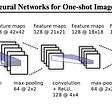## Feature size or Weight matrix size: Which one you should be worried about in DNN based object…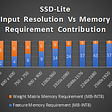## Training MXNet — part 1: MNIST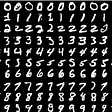## Review: PR-302-NeRF: Representing Scenes as Neural Radiance Fields for View Synthesis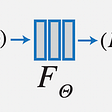## Unsupervised Clustering Algorithms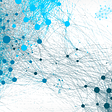## Chapter 1: The Machine Learning Landscape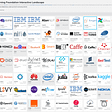## Distributed training in the cloud: Cloud Machine Learning Engine## Alibaba Cloud

Follow me to keep abreast with the latest technology news, industry insights, and developer trends. Alibaba Cloud website:https://www.alibabacloud.com

## Starting with TensorFlow Datasets -part 1; An intro to tf.datasets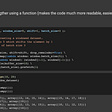## Optimizing a TensorFlow Input Pipeline: Best Practices in 2022## Phishing URL Detection Using ML